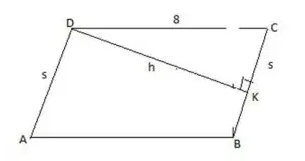### CAT 2018 – Slot 2 – Quantitative Ability – Ramesh and Ganesh can together complete a work in 16 days

Q. 1: Ramesh and Ganesh can together complete a work in 16 days. After seven days of working together, Ramesh got sick and his efficiency fell by 30%. As a result, they completed the work in 17 days instead of 16 days. If Ganesh had worked alone after Ramesh got sick, in how many days would he have completed the remaining work?
1. 13.5
2. 11
3. 12
4. 14.5

Let Ram do x unit of work each day, working with his full efficiency.

So work done by him in last ten days ( when his efficiency dropped by 30% ) = 0.7 *10x = 7x

Had he worked with his full efficiency he must have done 9x unit work in last 9 days , thus Ganesh must have done (9x -7x = 2x) work on 17th day.

Thus ratio of efficiency of Ram and Ganesh = 1:2

So total work = 16×(x+2x)=48x

Work remaining after 7 days = 48x-7×3x=27x

Time taken by Ganesh to complete this work = 27x/2x=13.5

##### Q. 2: A jar contains a mixture of 175 ml water and 700 ml alcohol. Gopal takes out 10% of the mixture and substitutes it by water of the same amount. The process is repeated once again. The percentage of water in the mixture is now1.   35.22.   30.33.   20.54.   25.4

initial volume of Alcohol = 700, total volume = 700+ 175 = 875 , replaced quantity = 87.5 & n =2

Using, final volume =initial volume ( 1-(replaced quantity)/(total volume))^n = 700(1-87.5/875)^2 = 700×81/100 = 567

So water in final mixture = 875 – 567 = 308

Required percentage = 308/875×100 = 35.2

Q. 3:  In a tournament, there are 43 junior level and 51 senior level participants. Each pair of juniors play one match. Each pair of seniors play one match. There is no junior versus senior match. The number of girl versus girl matches in junior level is 153, while the number of boy versus boy matches in senior level is 276. The number of matches a boy plays against a girl is

Among a group of n persons, number of matches played = n(n – 1)/2 Among the Junior participants, let the number of girls be n.

The number of matches played among girls

= n(n – 1)/2 = 153

=> n(n – 1) = 306 = 18 × 17 => n = 18

Number of boys = 43 – 18 = 25

The number of matches played between a boy and a girl = 25×18 = 450 Among the Senior level participants, let the number of boys be n.

The number of matches played between two boys

= n(n – 1)/2 = 276

=> n(n – 1) = 552 = 24 × 23 => n = 24

The number of girls = 51 – 24 = 27

The number of matches played between a boy and a girl = 27 × 24 = 648 Required answer = 450 + 648 = 1098

Q. 4: If A = {6^2n -35n -1: n = 1,2,3,…} and B = {35(n-1) : n = 1,2,3,…} then which of the following is true?
1. Neither every member of A is in B nor every member of B is in A
2. Every member of A is in B and at least one member of B is not in A
3. Every member of B is in A.
4.  At least one member of A is not in B

Given, A = 36^n – 35n – 1 = 36^n – 1^n – 35n

Since a^n – b^n is divisible by a – b for all positive integral values of n, So A is a

multiple of 35 for any integral value of n and B is a set containing all the

multiple of 35 including 0.

Hence, every member of A is in B but not every element of B is in A.

Q. 5: A parallelogram ABCD has area 48 sqcm. If the length of CD is 8 cm and that of AD is s cm, then which one of the following is necessarily true?
1. 5≤s≤7
2. s≤6
3. s≥6
4. s≠6As the area of ABCD = 48=s×h ————1)

In right-angled triangle CKD, DK ≤ CD ( CD is hypotenuses )

So h ≤8

Thus s ≥6——-(from eq 1)

##### Checkout Other Questions of CAT 2018 Slot 2 Paper:

Verbal Ability :              |   Q.01- Q.05  |  Q.06- Q.9  |  Q.10- Q.14  |  Q.15- Q.19  |  Q.20- Q.24  |  Q.25- Q.29  |  Q.30 – Q.34  |

Logical Reasoning :    |   Q.29 – Q.32  |

Quantitative Aptitude: |